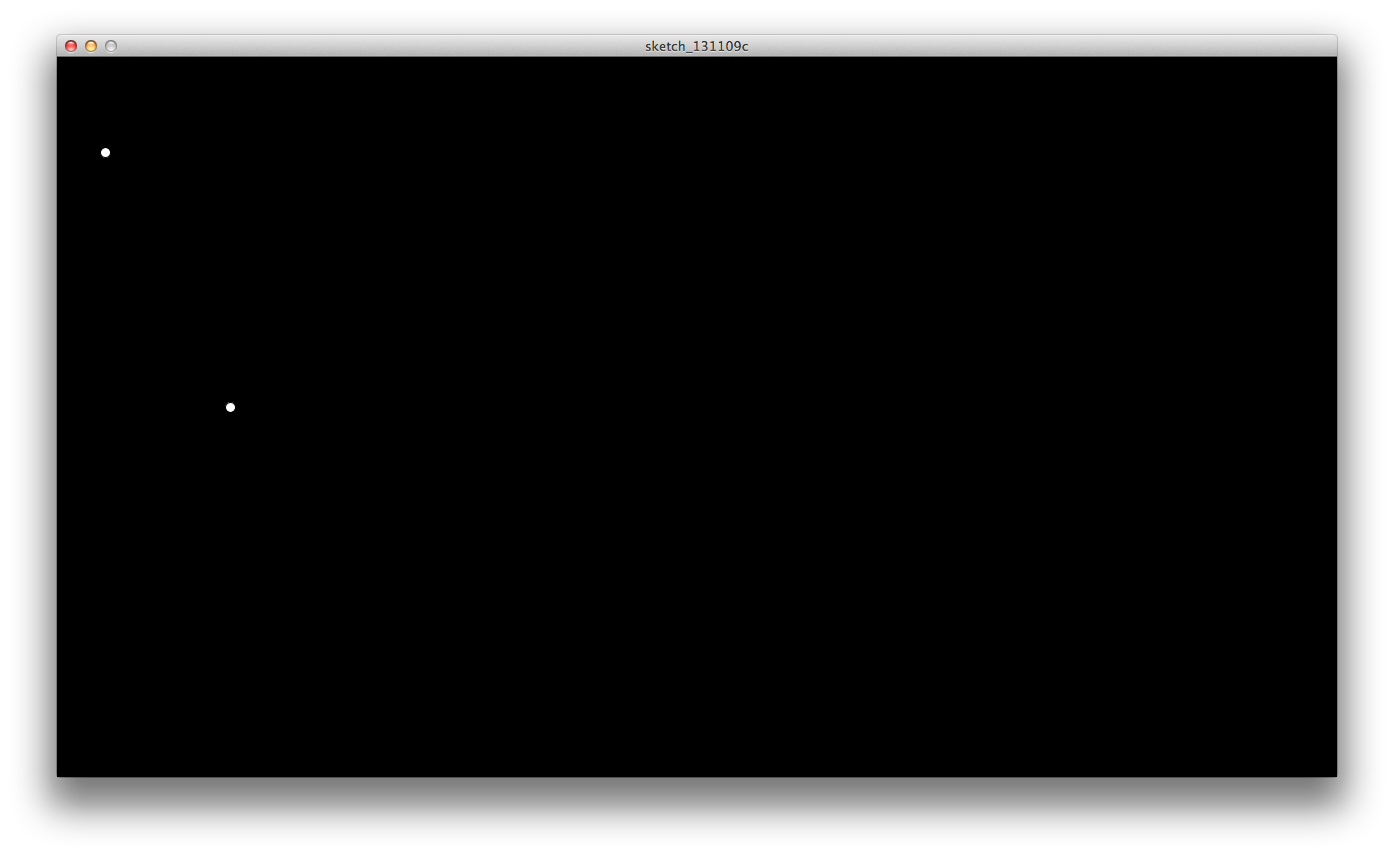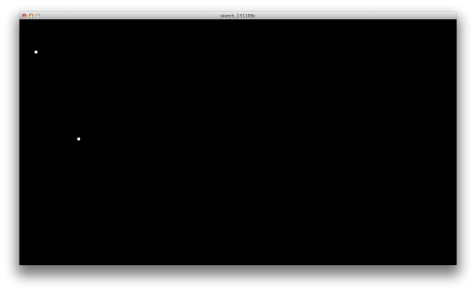# DataThis is my homework for the data assignment.
My idea was to show the change in the number of tweets about Lou Reed from during the week after his passing.
The dots representing each tweet would create Lou Reed’s name and fade with time, showing how a name appears and disappears in social media during a brief period of time.

Most of my time was spent on getting the data itself. I tried getting a Json from twitter and after struggling with it, opted for using scraperwiki.com  and got a CSV file with the tweets.

This is as far as I got with the sketch, which might be visually disappointing when comparing with the initial goal, but it involved a lot of learning about getting data and reading from it.This is my code:

Table dataset;
StringList allTime= new StringList();
float a;
float b;
float c;
float d;
float e;
float f;
void setup() {
size(1280,720);
a= random(0,100);
b= random (0,720);
c= random (100,200);
d= random (0,720);
e= random (200,300);
f= random (0,720);
smooth();

//read the data from the table

// add the column “time” to allTime stringlist
allTime.append(dataset.getStringColumn(“time”)); }

void draw(){
background(0);
if(allTime.hasValue(“10/30/13”) == true ) {
circle();
print(“yes”);

}

if(allTime.hasValue(“10/29/13”) == true ) {
circle2();
print(“29 “);

if(allTime.hasValue(“10/28/13”) == true ) {
circle3();
print(“28 “); }
}

}

void circle() {

ellipse(a,b,10,10);
fill(255);

}

void circle2(){
ellipse(c,d,10,10);
fill(255);

}

void circle3(){
ellipse(e,f,10,10);
fill(255);

}